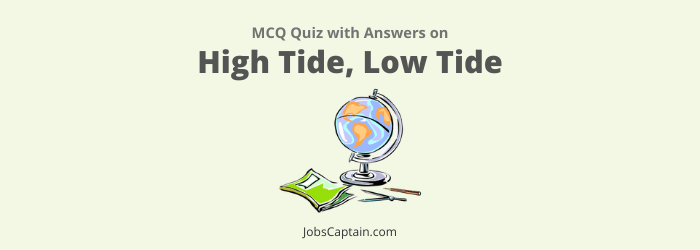# High Tide, Low Tide QuizQuestion 1. Spring Tide occurs_______.

(A) When the night is very cold

(B) When a strong wind blows

(C) When the Sun and Moon make a right angle

(D) When the Sun, the Earth and Moon are in a straight line

(D) When the Sun, the Earth and Moon are in a straight line

Question 2. The high tide in the Ocean is caused by________.

(A) Moon

(B) Stars

(C) Sun

(D) Earthquake

(A) Moon

Question 3. What are the causes of high-tide low-tide formation in the ocean?

(A) Due to Gravitation, Centripetal force and the Centrifugal force

(B) Due to the combined effect of Sun and the Moon

(C) Due to rotation of the Earth

(D) Due to the effect of the Sun

(A) Due to Gravitation, Centripetal force and the Centrifugal force

Question 4. The cause of producing indirect high tide is_______.

(A) Gravitational force of the Earth

(B) Centrifugal force of the Earth

(C) Gravitational force of the Sun

(D) Gravitational force of the Moon

(B) Centrifugal force of the Earth

Question 5. Tides occurs in oceans and seas due to which among the following?
1. Gravitational force of Sun.
2. Gravitational force of Moon.
3. Centrifugal force of Earth.
Select the correct answer using the code given below.

(A) 1, 2 and 3

(B) Only 1 and 3

(C) Only 2 and 3

(D) Only 1

(A) 1, 2 and 3

Question 6. Statement (A): During the times of neap-tide, high-tide is below normal and low-tide is above normal.
Reason (R): Neap-tides occurs during the new-Moon instead of full-Moon.
Choose the correct answer using following options.

(A) (A) is false, but (R) is true

(B) (A) is true, but (R) is false

(C) Both (A) and (R) are true, but (R) is not the correct explanation of (A)

(D) Both (A) and (R) are true and (R) is the correct explanation of (A)